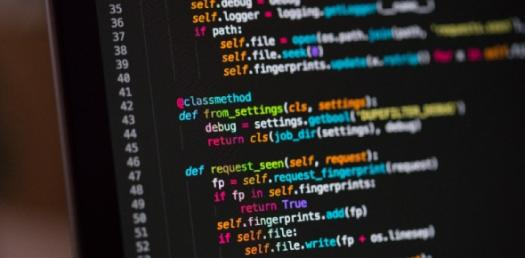# Programming: Python Quiz

17 Questions | Attempts: 2768
ShareSettings• 1.
Python writes data on screen using which function?
• A.

Cout

• B.

(display )

• C.

Print()

• D.

Echo

• E.

Console.log()

• F.

System.out.println()

• 2.
Python can recognise this data: "hello"
• A.

Yes

• B.

No

• C.

Sometimes

• 3.
Python can recognise this data: 56.97
• A.

Yes

• B.

No

• C.

Sometimes

• 4.
Python can recognise this data: computer
• A.

Yes

• B.

No

• C.

Sometimes

• 5.
Python can recognise this code: 3 + 6 * 8
• A.

Yes

• B.

No

• C.

Sometimes

• 6.
What does Python use to store values?
• 7.
Python will run this program without errors: print("hello")
• A.

True

• B.

False

• 8.
Python will run this program without errors: print(765.9908) print("that was a number")
• A.

True

• B.

False

• 9.
The output of this program will be? print("5+7+2")
• A.

14

• B.

12

• C.

5+7+2

• D.

Nothing. It will return an error.

• 10.
What will the output of this program be? print("Hello") text = "This is a number" print("Hi, " + text) number = 4 print(box + 2) print(box * 2) print(box * box - box)
• A.

Hello Hi, This is a number 6 8 12

• B.

HelloHi, This is a number6812

• C.

Nothing, it will return an error

• D.

Hello Hi, text box2 boxbox box

• E.

Hello This is a number Hi, 4 box2 boxbox boxbox-box

• 11.
This program will run without errors: print(x) x = 3 print(x+3)
• A.

True

• B.

False

• 12.
What structure do you use to let the computer make decisions in Python?
• A.

Because structure

• B.

If statement

• C.

Decision structure

• D.

Decide statement

• 13.
What optional part can you add to an if statement?
• A.

Who

• B.

Else

• C.

Then

• D.

Nothing

• 14.
Which of these could python run without errors?
• A.

X = 5 if x < 10 print("hi")

• B.

X = 5 if x < 10: print("hi")

• C.

Both

• D.

Neither

• 15.
This program is full of errors. What do you need to change to make it correct?cat_weight = 45 if cat_wieght = 50; print your cat is healthy else: print unhealthy
• A.

The variable name has been spelled wrong

• B.

• C.

If statements end in a colon, not semicolon

• D.

Instructions underneath an if and an else should be indented by 4 spaces

• E.

Every line ends in a semicolon

• F.

Words that python should understand should be "surrounded by quotes".

• G.

You have to say what type of data something is when you declare a variable.

• H.

Else statements end in a semicolon, not a colon

• I.

Print() should always be written with brackets

• J.

You should use ==, not = to check if two things are equal

• K.

You should always include units when working with numbers in Python

## Related TopicsBack to top
×

Wait!
Here's an interesting quiz for you.